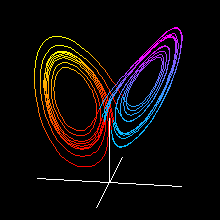Lorenz Attractor AnimationThe transparent version of this animation is here.

Let us consider the dynamics generated by the following differential equations.

 dx/dt= -10x +10y dy/dt= 28x -y -xz dz/dt= -8/3z +xy

The dynamics of the system converge to a strange attractor called Lorenz attractor.
By rotating the viewpoint around the attractor, the animation in this page is obtained.
The dynamics of the trajectory are also observed in "Lorenz attractor" simulator.

<< Duffing Equation Animation

Introduction to Chaos and Nonlinear Dynamics